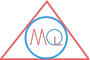# Famous Mathematicians

## Al Khawarizmi

Born 780 CE After 847 Arithmetic, algebra, astronomy

Al Khawarizmi was a Persian mathematician who worked in the fields of mathematics, astronomy and geography. He has been named as the father of algebra. He was responsible for spreading the Hindu-Arabic Numeral system to the Middle East and Europe.

## Archimedes

Born 287 BC 212 BC Syracuse, Italy Archimedes Principle

Archimedes was a Greek mathematician and astronomer from the city of Syracuse. He is considered as one of the greatest mathematician of all the time. Archimedes worked on many geometrical theorems like the area of circle, surface area and volume of sphere, area of an ellipse, area under a parabola and the area of spiral. His very famous Archimedes Principle has been universally accepted.

## Aryabhata

Born 476 CE 550 CE Pataliputra, Gupta Empire

Aryabhata was an Indian mathematician and astronomer in Gupta era. He worked on topics such as finding of square and cube roots, mensuration, arithmetic progression, quadratic equations, linear and indeterminate equations. He calculated the value of $$\pi$$ upto 4 decimal places.

## Blaise Pascal

Born 19 June 1623 19 August 1662 Paris, France Probability, Conic sections Pascal triangle

Blaise Pascal was a French mathematician in the 17th century. He worked on Probability and conic sections when he was teenager only. Later he introduced a tabular representation of binomial coefficients which is now known as pascal’s triangle.

## Carl Freidrich Gauss

Born 30 April 1777 23 February 1855 Brunswick, Germany

Carl Freidrich Gauss was a German mathematician and considered as the most influential mathematicians of the history. He started solving many problems of maths since his early childhood. Many terms in algebra, geometry, number theory and physics are after his name.

## Euclid

Born 325 BCE 265 BCE Greece Geometry Elements

Euclid was of Greek origin and is also known as father of geometry. Euclid’s birthdate is unknown and 325 BCE – 265 BCE is an estimated time period of his life. He is one of the most influential mathematician in history of mathematics due to his written work on fundamentals of geometry in Elements.

## Fancios Vitea

Born 1540 23 February 1603 France ViÃ¨te’s formula

Fancios Vitea was a French mathematician who worked on algebra who first created symbolic algebra. He was a lawyer by trade though.

## George Rheticus

Born 16 February 1514 4 December 1574 Austria Trigonometry

George Rheticus was born in Austria and is known for his trigonometric tables.

## Heron or Hero of Alexandria

Hero of Alexendria is also known as Heron of Alexendria and was Greek mathematician and worked in the field of physics also. He worked most of his life in his native city Alexendria. He is remembered for his known Heron’s formula to find the area of a triangle from length of its sides.

## Pierre De Fermat

Born 1607 12 January, 1665 France Number Theory Fermat’s last theorem

Pierre De Fermat was a French mathematician who researched into the number theory and has played a significant role in early developments of infinitesimal calculus. He is known for his Fermat’s last theorem in number theory.

## Pierre Simon Laplace

Born 23 March 1749 5 March 1827 France Laplace transform

Pierre Simon Laplace was a French mathematician and a polymath who worked in the fields of mathematics, statistics, physics, astronomy and philosophy. He formulated Laplace’s equation and have been famous for his Laplace transform. He is honored as the greatest scientist of all time.

## Pythagoras

Born 495 BC 510 BC Greece Mathematics Pythagoras Theorem

Pythagoras was a Greek philosopher and known abouts his life are ambiguous but as per little knowledge to known his birth place is Samos. He is well remembered for his historical discovery in mathematics and is famous for Pythagoras Theorem.

## Rene Descartes

Born 31 March 1596 11 February 1650 France Geometry and Algebra

Rene Descartes was a French mathematician, a philosopher and scientist. He introduced Cartesian geometry or Coordinate geometry which is a widely used branch in mathematics.

## Srinivasa Ramanujan

Born 22 December 1887 26 April 1920 India

Srinivasa Ramanujan was an Indian mathematician who contributed to number theory, infinite series and continued fractions in his short span of life only. He was the youngest to receive Fellows of the Royal Society and elected as Fellow of Trinity College, Cambridge.

## Thales of Miletus

Born 626 BC 548 BC Greece Geometry Thales Theorem

Thales of Miletus was a Greek mathematician, astronomer and is known as Father of Science. He is much famous for his theorems that the diameter of a circle subtends a right angle at any point on the circle and the well known Basic Proportionality Theorem.